# RD Sharma Solutions for Class 9 Maths Chapter 20 Surface Area and Volume of A Right Circular Cone Exercise 20.2

This exercise solutions will help students understand the chapter in a much better way. The solutions will further enable them to study at their own pace in a much more streamlined manner. RD Sharma Class 9 solutions contain answers to all the exercise questions which will make studying this unit easy. Students can also increase their question-solving efficiency by referring to the solutions.

## Download PDF of RD Sharma Solutions for Class 9 Maths Chapter 20 Surface Area and Volume of A Right Circular Cone Exercise 20.2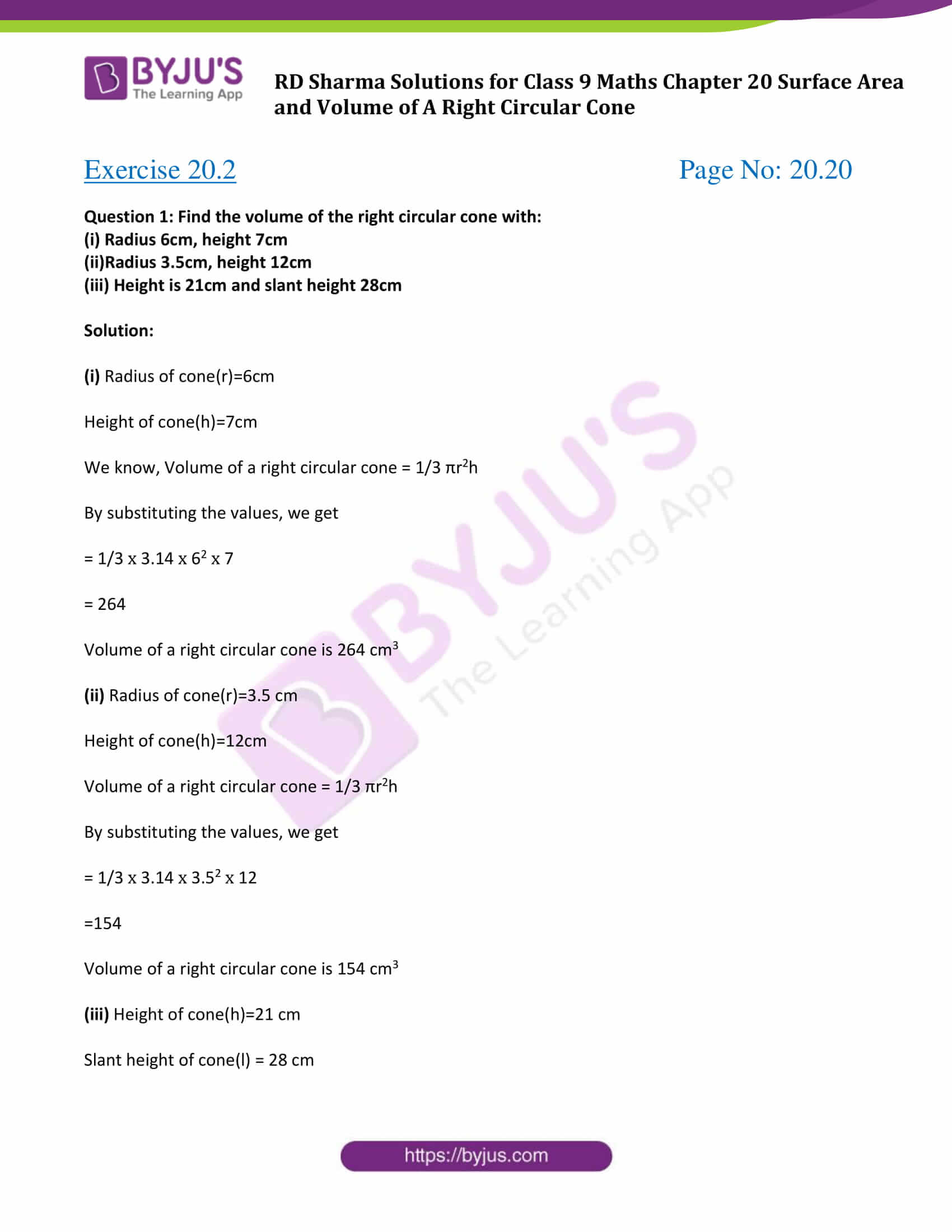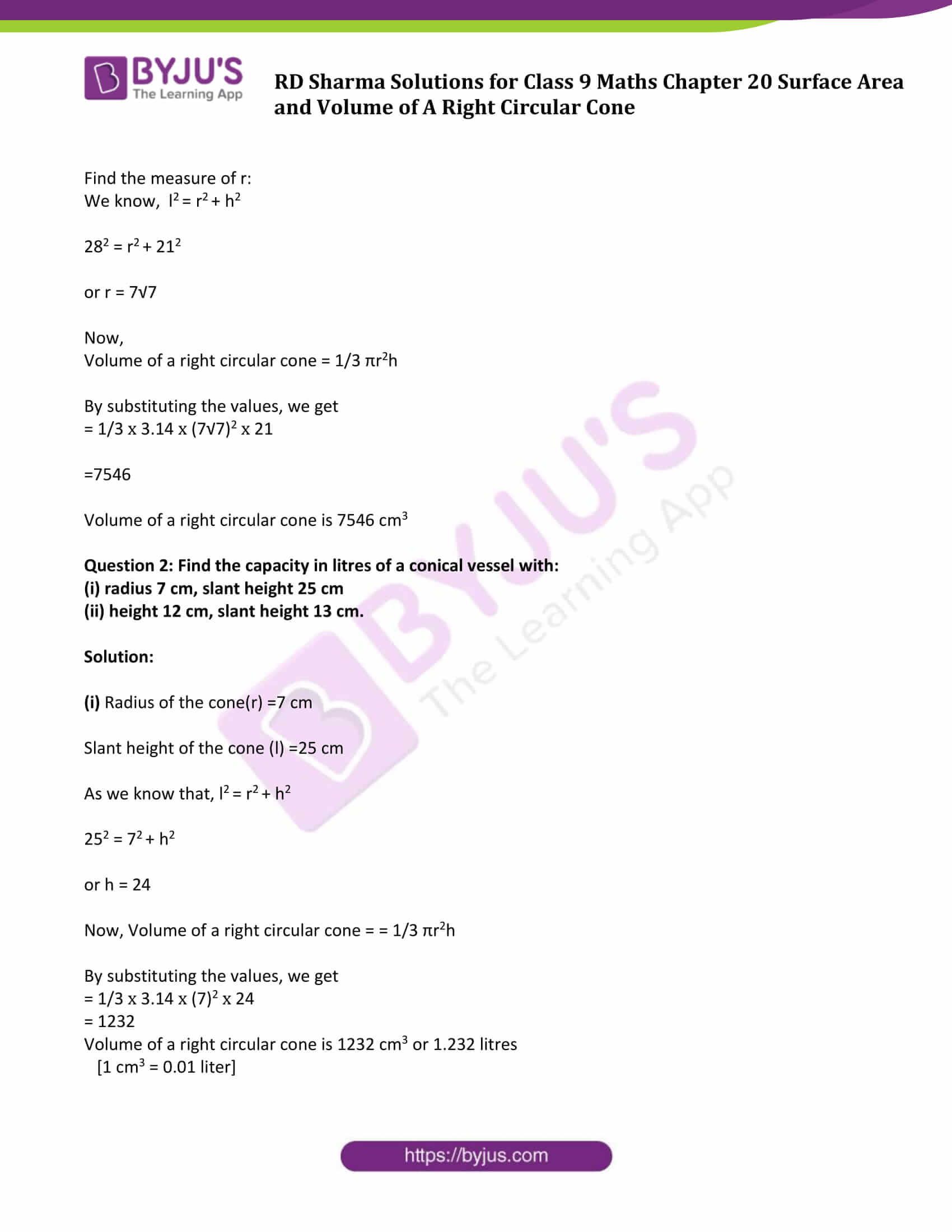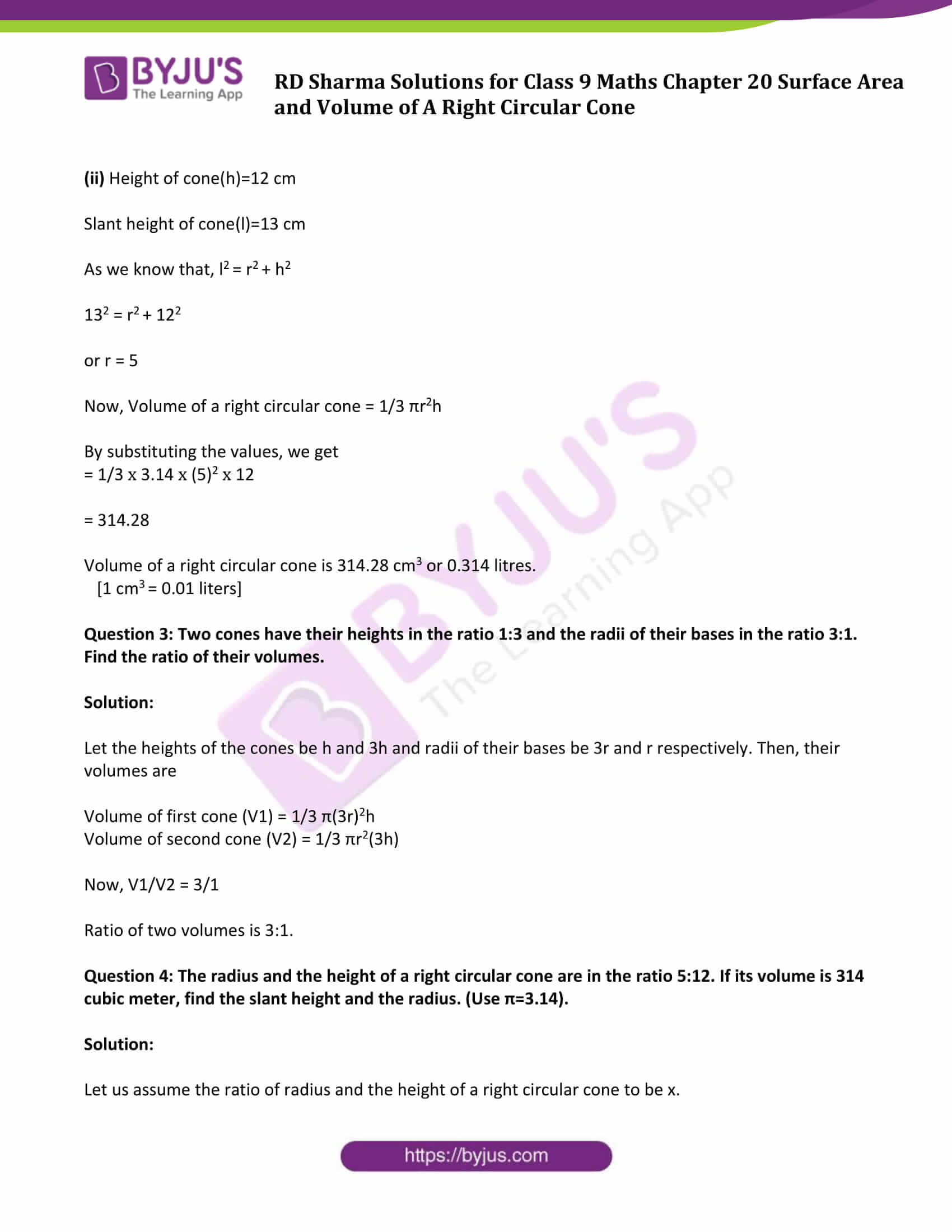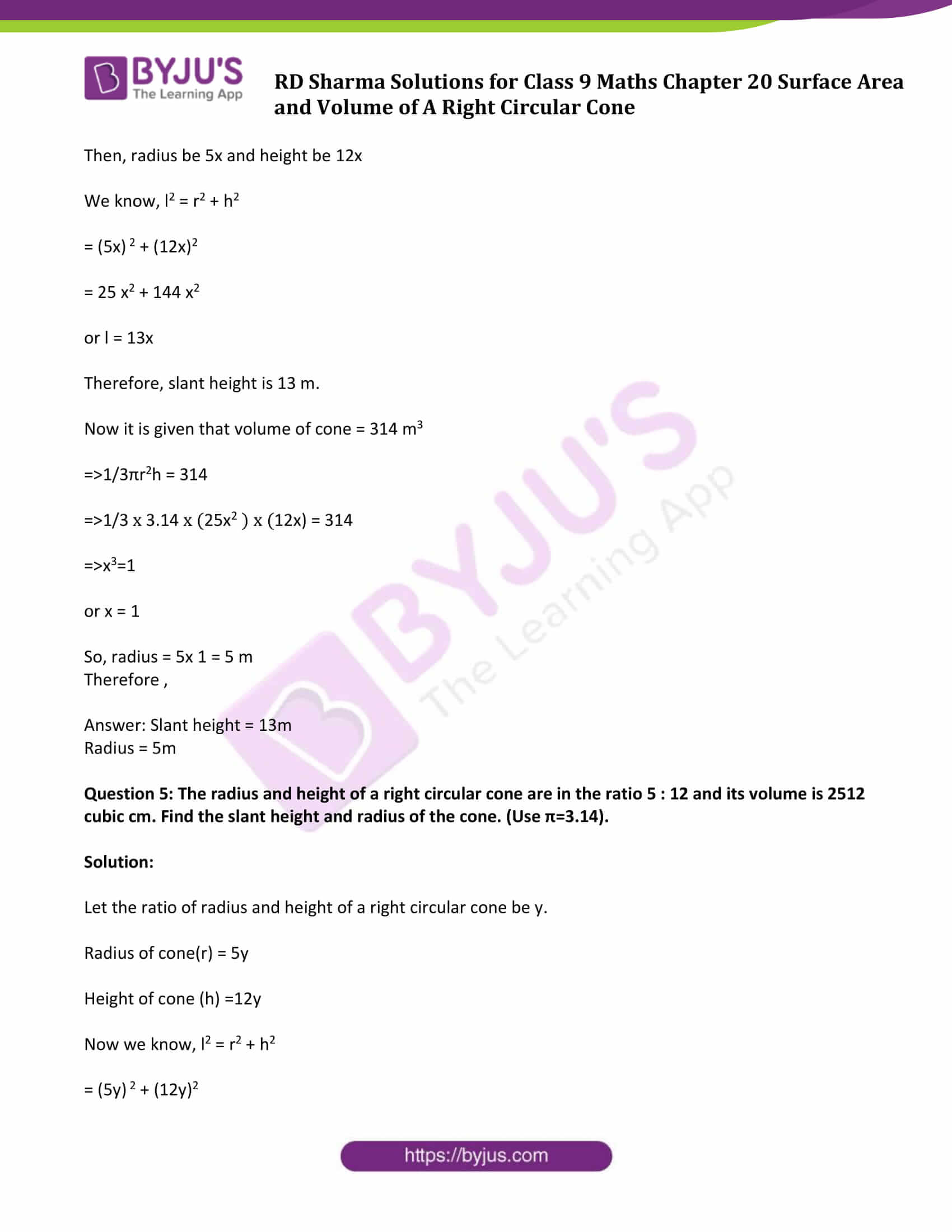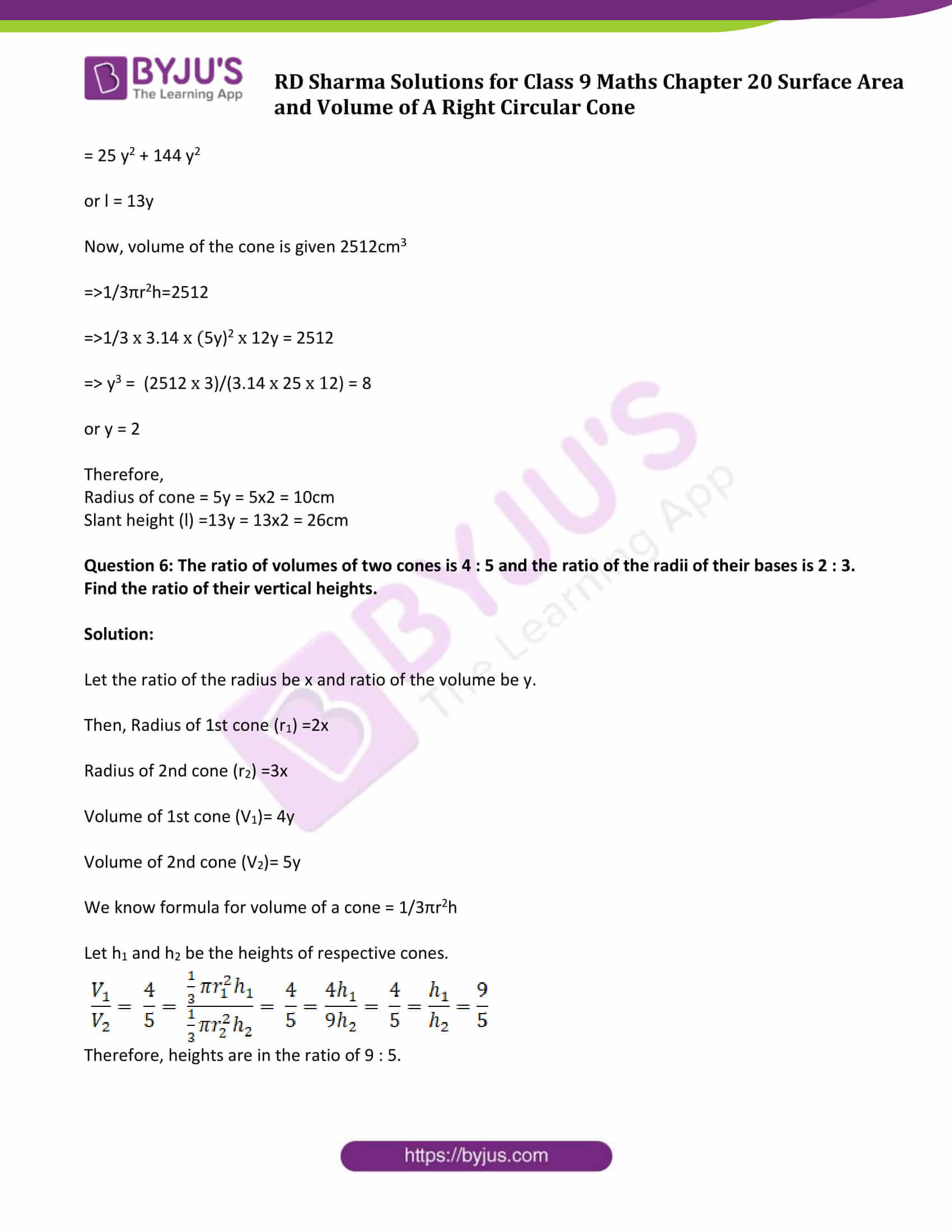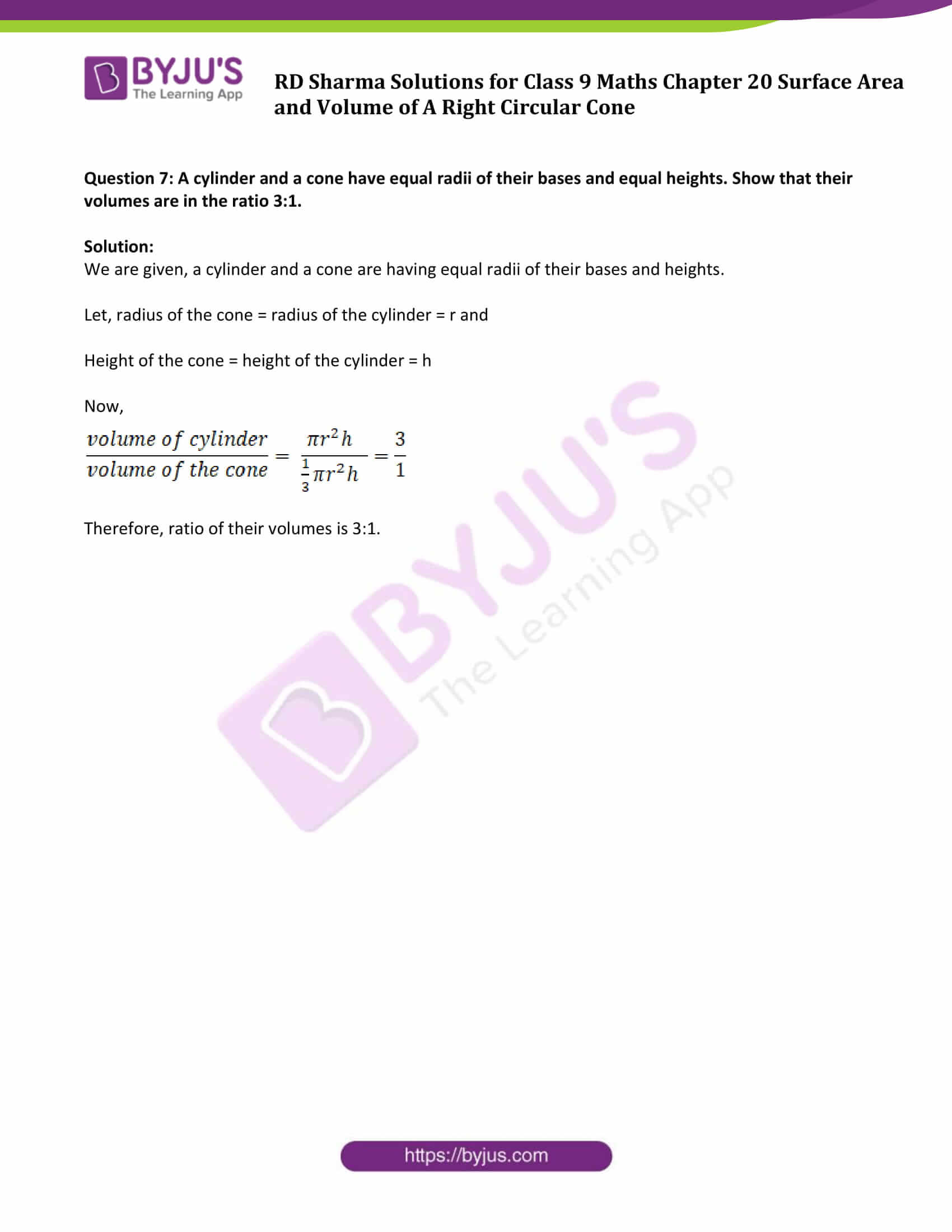### Access Answers to Maths RD Sharma Solutions for Class 9 Chapter 20 Surface Area and Volume of A Right Circular Cone Exercise 20.2 Page number 20.20

Question 1: Find the volume of the right circular cone with:

(iii) Height is 21cm and slant height 28cm

Solution:

Height of cone(h)=7cm

We know, Volume of a right circular cone = 1/3 πr2h

By substituting the values, we get

= 1/3 x 3.14 x 62 x 7

= 264

Volume of a right circular cone is 264 cm3

Height of cone(h)=12cm

Volume of a right circular cone = 1/3 πr2h

By substituting the values, we get

= 1/3 x 3.14 x 3.52 x 12

=154

Volume of a right circular cone is 154 cm3

(iii) Height of cone(h)=21 cm

Slant height of cone(l) = 28 cm

Find the measure of r:

We know, l2 = r2 + h2

282 = r2 + 212

or r = 7√7

Now,

Volume of a right circular cone = 1/3 πr2h

By substituting the values, we get

= 1/3 x 3.14 x (7√7)2 x 21

=7546

Volume of a right circular cone is 7546 cm3

Question 2: Find the capacity in litres of a conical vessel with:

(i) radius 7 cm, slant height 25 cm

(ii) height 12 cm, slant height 13 cm.

Solution:

(i) Radius of the cone(r) =7 cm

Slant height of the cone (l) =25 cm

As we know that, l2 = r2 + h2

252 = 72 + h2

or h = 24

Now, Volume of a right circular cone = = 1/3 πr2h

By substituting the values, we get

= 1/3 x 3.14 x (7)2 x 24

= 1232

Volume of a right circular cone is 1232 cm3 or 1.232 litres

[1 cm3 = 0.01 liter]

(ii) Height of cone(h)=12 cm

Slant height of cone(l)=13 cm

As we know that, l2 = r2 + h2

132 = r2 + 122

or r = 5

Now, Volume of a right circular cone = 1/3 πr2h

By substituting the values, we get

= 1/3 x 3.14 x (5)2 x 12

= 314.28

Volume of a right circular cone is 314.28 cm3 or 0.314 litres.

[1 cm3 = 0.01 liters]

Question 3: Two cones have their heights in the ratio 1:3 and the radii of their bases in the ratio 3:1. Find the ratio of their volumes.

Solution:

Let the heights of the cones be h and 3h and radii of their bases be 3r and r respectively. Then, their volumes are

Volume of first cone (V1) = 1/3 π(3r)2h

Volume of second cone (V2) = 1/3 πr2(3h)

Now, V1/V2 = 3/1

Ratio of two volumes is 3:1.

Question 4: The radius and the height of a right circular cone are in the ratio 5:12. If its volume is 314 cubic meter, find the slant height and the radius. (Use π=3.14).

Solution:

Let us assume the ratio of radius and the height of a right circular cone to be x.

Then, radius be 5x and height be 12x

We know, l2 = r2 + h2

= (5x) 2 + (12x)2

= 25 x2 + 144 x2

or l = 13x

Therefore, slant height is 13 m.

Now it is given that volume of cone = 314 m3

⇒ 1/3πr2h = 314

⇒ 1/3 x 3.14 x (25x2 ) x (12x) = 314

⇒ x3=1

or x = 1

So, radius = 5x 1 = 5 m

Therefore ,

Question 5: The radius and height of a right circular cone are in the ratio 5 : 12 and its volume is 2512 cubic cm. Find the slant height and radius of the cone. (Use π=3.14).

Solution:

Let the ratio of radius and height of a right circular cone be y.

Height of cone (h) =12y

Now we know, l2 = r2 + h2

= (5y) 2 + (12y)2

= 25 y2 + 144 y2

or l = 13y

Now, volume of the cone is given 2512cm3

⇒ 1/3πr2h=2512

⇒ 1/3 x 3.14 x (5y)2 x 12y = 2512

⇒ y3 = (2512 x 3)/(3.14 x 25 x 12) = 8

or y = 2

Therefore,

Radius of cone = 5y = 5×2 = 10cm

Slant height (l) =13y = 13×2 = 26cm

Question 6: The ratio of volumes of two cones is 4 : 5 and the ratio of the radii of their bases is 2 : 3. Find the ratio of their vertical heights.

Solution:

Let the ratio of the radius be x and ratio of the volume be y.

Then, Radius of 1st cone (r1) =2x

Radius of 2nd cone (r2) =3x

Volume of 1st cone (V1)= 4y

Volume of 2nd cone (V2)= 5y

We know formula for volume of a cone = 1/3πr2h

Let h1 and h2 be the heights of respective cones.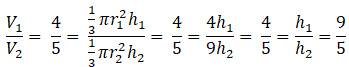Therefore, heights are in the ratio of 9 : 5.

Question 7: A cylinder and a cone have equal radii of their bases and equal heights. Show that their volumes are in the ratio 3:1.

Solution:

We are given, a cylinder and a cone are having equal radii of their bases and heights.

Let, radius of the cone = radius of the cylinder = r and

Height of the cone = height of the cylinder = h

Now,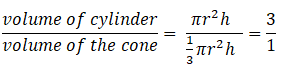Therefore, ratio of their volumes is 3:1.

## RD Sharma Solutions for Class 9 Maths Chapter 20 Surface Area and Volume of A Right Circular Cone Exercise 20.2

RD Sharma Solutions Class 9 Maths Chapter 20 Surface Area and Volume of A Right Circular Cone Exercise 20.2 is based on topic – Volume of a Right Circular Cone. RD Sharma class 9 solutions is one of the best tools to practice Maths concepts and get ready for exams.